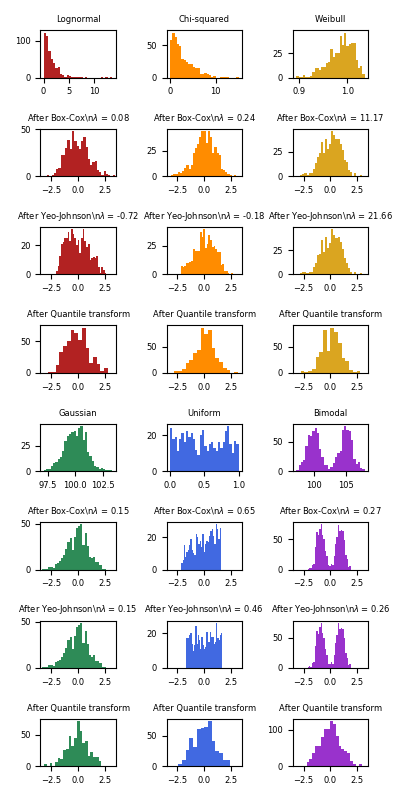# Map data to a normal distribution¶

This example demonstrates the use of the Box-Cox and Yeo-Johnson transforms through preprocessing.PowerTransformer to map data from various distributions to a normal distribution.

The power transform is useful as a transformation in modeling problems where homoscedasticity and normality are desired. Below are examples of Box-Cox and Yeo-Johnwon applied to six different probability distributions: Lognormal, Chi-squared, Weibull, Gaussian, Uniform, and Bimodal.

Note that the transformations successfully map the data to a normal distribution when applied to certain datasets, but are ineffective with others. This highlights the importance of visualizing the data before and after transformation.

Also note that even though Box-Cox seems to perform better than Yeo-Johnson for lognormal and chi-squared distributions, keep in mind that Box-Cox does not support inputs with negative values.

For comparison, we also add the output from preprocessing.QuantileTransformer. It can force any arbitrary distribution into a gaussian, provided that there are enough training samples (thousands). Because it is a non-parametric method, it is harder to interpret than the parametric ones (Box-Cox and Yeo-Johnson).

On “small” datasets (less than a few hundred points), the quantile transformer is prone to overfitting. The use of the power transform is then recommended.# Author: Eric Chang <ericchang2017@u.northwestern.edu>
#         Nicolas Hug <contact@nicolas-hug.com>

import numpy as np
import matplotlib.pyplot as plt

from sklearn.preprocessing import PowerTransformer
from sklearn.preprocessing import QuantileTransformer
from sklearn.model_selection import train_test_split

print(__doc__)

N_SAMPLES = 1000
FONT_SIZE = 6
BINS = 30

rng = np.random.RandomState(304)
bc = PowerTransformer(method='box-cox')
yj = PowerTransformer(method='yeo-johnson')
# n_quantiles is set to the training set size rather than the default value
# to avoid a warning being raised by this example
qt = QuantileTransformer(n_quantiles=500, output_distribution='normal',
random_state=rng)
size = (N_SAMPLES, 1)

# lognormal distribution
X_lognormal = rng.lognormal(size=size)

# chi-squared distribution
df = 3
X_chisq = rng.chisquare(df=df, size=size)

# weibull distribution
a = 50
X_weibull = rng.weibull(a=a, size=size)

# gaussian distribution
loc = 100
X_gaussian = rng.normal(loc=loc, size=size)

# uniform distribution
X_uniform = rng.uniform(low=0, high=1, size=size)

# bimodal distribution
loc_a, loc_b = 100, 105
X_a, X_b = rng.normal(loc=loc_a, size=size), rng.normal(loc=loc_b, size=size)
X_bimodal = np.concatenate([X_a, X_b], axis=0)

# create plots
distributions = [
('Lognormal', X_lognormal),
('Chi-squared', X_chisq),
('Weibull', X_weibull),
('Gaussian', X_gaussian),
('Uniform', X_uniform),
('Bimodal', X_bimodal)
]

colors = ['firebrick', 'darkorange', 'goldenrod',
'seagreen', 'royalblue', 'darkorchid']

fig, axes = plt.subplots(nrows=8, ncols=3, figsize=plt.figaspect(2))
axes = axes.flatten()
axes_idxs = [(0, 3, 6, 9), (1, 4, 7, 10), (2, 5, 8, 11), (12, 15, 18, 21),
(13, 16, 19, 22), (14, 17, 20, 23)]
axes_list = [(axes[i], axes[j], axes[k], axes[l])
for (i, j, k, l) in axes_idxs]

for distribution, color, axes in zip(distributions, colors, axes_list):
name, X = distribution
X_train, X_test = train_test_split(X, test_size=.5)

# perform power transforms and quantile transform
X_trans_bc = bc.fit(X_train).transform(X_test)
lmbda_bc = round(bc.lambdas_, 2)
X_trans_yj = yj.fit(X_train).transform(X_test)
lmbda_yj = round(yj.lambdas_, 2)
X_trans_qt = qt.fit(X_train).transform(X_test)

ax_original, ax_bc, ax_yj, ax_qt = axes

ax_original.hist(X_train, color=color, bins=BINS)
ax_original.set_title(name, fontsize=FONT_SIZE)
ax_original.tick_params(axis='both', which='major', labelsize=FONT_SIZE)

for ax, X_trans, meth_name, lmbda in zip(
(ax_bc, ax_yj, ax_qt),
(X_trans_bc, X_trans_yj, X_trans_qt),
('Box-Cox', 'Yeo-Johnson', 'Quantile transform'),
(lmbda_bc, lmbda_yj, None)):
ax.hist(X_trans, color=color, bins=BINS)
title = 'After {}'.format(meth_name)
if lmbda is not None:
title += r'\n$\lambda$ = {}'.format(lmbda)
ax.set_title(title, fontsize=FONT_SIZE)
ax.tick_params(axis='both', which='major', labelsize=FONT_SIZE)
ax.set_xlim([-3.5, 3.5])

plt.tight_layout()
plt.show()


Total running time of the script: ( 0 minutes 1.536 seconds)

Gallery generated by Sphinx-Gallery